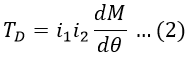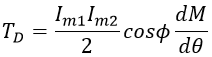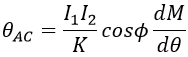# Electrodynamometer Type Wattmeter - Construction, Working & Errors

It is a transfer type instrument that is calibrated with dc but can be used for ac measurements without any changes. The electrodynamic instruments are often used in ac voltmeters and ammeters but they can be used as wattmeters with slight changes. Let us see the construction and working principle of the electrodynamometer type wattmeter.

## Construction of Electrodynamometer Type Wattmeter :

The constructional details of an electro-dynamometer type wattmeter are shown in the below figure.

### Fixed Coils :

It is basically an air-cored coil with a large area of cross-section and fewer turns. The fixed coils are divided into two sections and a moving coil is placed between them. These two coils are connected in series with line and hence carry the full line current. The main function of the fixed coil is to produce the required flux. The field coils are laminated and varnished as they carry heavy current and they are also known as current coils.

### Moving Coil :

The moving coil is placed between the two fixed coils and made to rotate with the help of a spindle. A pointer is attached to the spindle that moves with the moving coil over the scale. The coil is wounded on a metallic former and connected across the line. It is also known as the voltage coil or pressure coil.

The moving coil is made with a small cross-sectional area and more turns so as to limit the current through it. The resistance of the voltage coil can be increased by connecting a resistance in series with it.

### Springs :

Since the moving coil is not a stationary element, it cannot be connected directly to the supply. The supply to the moving coil is given through springs. These springs also provide the necessary controlling torque to control the deflection of the pointer.

### Dampers :

This type of instrument is provided with air friction damping to damp out oscillations by providing necessary damping torque. The moving coils carry a pair of aluminum vanes, which move in sector shaped chamber at the bottom of the instrument.

### Shielding :

The field produced by this instrument is very weak and hence may get affected easily by the stray magnetic field. Hence, in order to overcome this drawback shielding is done in the form of casing of high permeability.

## Working of Electrodynamometer Type Wattmeter :

The fixed coil connected in series acts as a current coil and the moving coil connected across the supply terminals acts as a voltage coil. The fixed coil is proportional to the load current and the moving coil is proportional to the voltage.

When the current passes through these coils, the fixed coil, as well as the moving coil, creates a magnetic field due to which, the deflecting torque is developed, which is proportional to power. Thus, the power can be measured by using the electrodynamic type wattmeter.

### Expression for Torque :

#### Let,ip = Instantaneous current in the fixed coilic = Instantaneous current in moving coilM = Mutual inductance between fixed and working coilT = Time periodTI = Instantaneous torqueTD = Deflecting torqueThe deflecting torque is given by,But, the instantaneous torque is given by,Substituting equation 2 in 1, we get,Assume, the two currents i1 and i2 to be sinusoidal with a phase difference of Φ we get, i1 = Im1 sinωti2 = Im2 sin(ωt - Φ) Substituting, i1 and i2 values in equation 3, we get,On solving equation 4, we get,In dc operation of a dynamometer type wattmeter, the deflection produced in the torque is given by,Where,I1, I2 = Currents in fixed and moving coils respectivelydM/dθ = Rate of change of mutual inductancek = Spring constant In ac operation of a dynamometer type wattmeter, the deflection produced in the torque is given by,Where,I1, I2 = RMS values of currents in fixed and moving coils respectivelycos Φ = Power factor

Hence, the deflection is proportional to the product of RMS values of the currents in both coils, power factor, and rate of change of mutual inductance.

Therefore, for a dynamometer-type wattmeter, the scale is calibrated with the square root of the current that is squared, which is nothing but the RMS value of the ac quantity. Hence, in this way, both ac and dc can be measured using dynamometer type wattmeter and indicates power whether it is connected in ac or dc circuit.

## Errors in Electrodynamometer Type Wattmeter :

### Advantages of Electrodynamometer Type Wattmeter :

#### A high degree of accuracy in the readings can be obtained.The scale of the instrument is uniform.Can be used in both ac and dc circuits.Due to the good accuracy of the instrument, it can be used for calibration.

Do not enter any spam links and messages